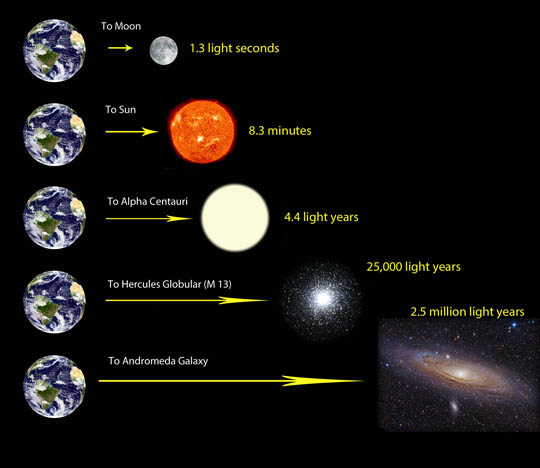Concepts

Class 8
Chapter 17 Class 8 - Stars and the Solar System

The distance between the stars is so large that Kilometers becomes a very small unit.

Hence, the distance between stars and planets are expressed in light years.

One Light Year means Distance travelled by light in 1 Year.

Calculating 1 Light Year

Speed of Light = 3 × 10 8 m/s

Time = 1 Year

= 365 days

= 365 × 24 hours

= 31536000 seconds

Now,

1 Light Year = Speed × Time

= 3 × 10 8 × 31536000 m

= 94608000 × 10 8 m

= 94608000 × 10 8 × 1/000 km

= 94608 × 10 8 km

= 9.4608 × 10 4 × 10 8 km

= 9.4608 × 10 12 km

## How much time will it take for light to reach from sun to earth?

Distance of sun to earth = 150000000 km

Speed of Light = 300000 km/second

Time taken = 150000000/300000 seconds

= 500 seconds

Time taken in minutes = 500 second/60

= 8.33 minutes

So, it takes around 8 minutes for Sunlight to reach earth

The next closest star is Proxima Centauri, 4.3 light years away.

So, if we see a light from that star today. It means the light we are seeing was actually 4.3 years agoNote: Light year is a unit of distance. Not time.

Learn in your speed, with individual attention - Teachoo Maths 1-on-1 Class